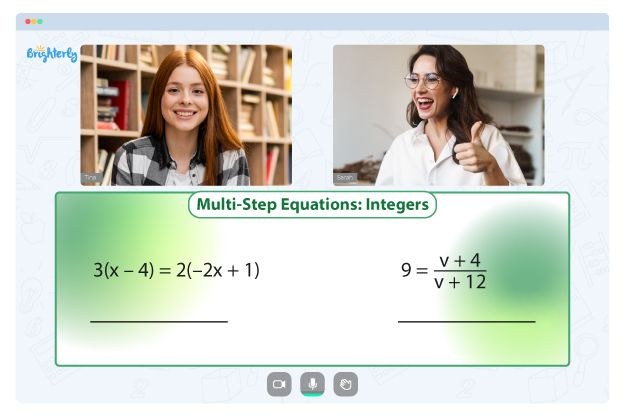# Solving Logarithmic Equations Worksheets

Logarithmic equations worksheets are a great way for kids to practice their math skills and develop problem-solving abilities. These worksheets are designed to help students understand and solve simple logarithmic equations, which are equations that involve logarithms.

The solving logarithmic equations worksheet includes a variety of problems that cover different types of logarithmic equations. These problems range from simple equations to more complex ones, and they are accompanied by a key. The solving log equations worksheet key provides step-by-step solutions to each problem.

### Benefits of Solving Logarithmic Equations Worksheets

One of the main benefits of using solving logarithmic equations worksheets is that they help students build their confidence in solving simple logarithmic equations. By practicing with these worksheets, students can become more familiar with the concept of logarithms and gain a better understanding of how they are used in equations.

Moreover, the solving logarithmic equations worksheet PDF format makes it easy for parents to download and print the worksheets at home. The worksheet also includes answers. Solving logarithmic equations worksheet answers makes it easy for parents to check their child’s work and help them if they get stuck.

Math for Kids

Is Your Child Struggling With Math?
1:1 Online Math TutoringSolving logarithmic equations worksheets are an excellent resource for parents who want to help their children develop their math skills. These worksheets are a fun and engaging way for students to learn about logarithmic equations and develop their problem-solving abilities. Download the solving logarithmic equations PDF for free to help your child improve their math skills.### Solving Logarithmic Equations Worksheet PDF

Solving Logarithmic Equations Worksheet### Solving Simple Logarithmic Equations PDF

Solving Simple Logarithmic Equations### Solving Logarithmic Equations PDF

Solving Logarithmic Equations PDF### Solving Log Equations Worksheet Key PDF

Solving Log Equations Worksheet Key

Struggling with Equations?• Does your child need extra assistance with understanding equations?
• Start learning with an online tutor.

Is your child finding it hard to master equations? An online tutor could be of help.